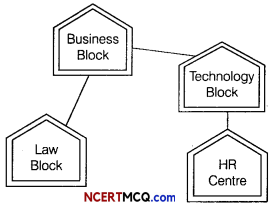Students can access the CBSE Sample Papers for Class 12 Computer Science with Solutions and marking scheme Term 2 Set 11 will help students in understanding the difficulty level of the exam.

## CBSE Sample Papers for Class 12 Computer Science Term 2 Set 11 with Solutions

Maximum Marks: 35
Time: 2 hours

Instructions:

• The question paper is divided into 3 sections – A, B and C.
• Section A, consists of 7 questions (1-7). Each question carries 2 marks.
• Section B, consists of 3 questions (8-10). Each question carries 3 marks.
• Section C, consists of 3 questions (11-13). Each question carries 4 marks.
• Internal choices have been given for question numbers 7, 8 and 12.

Section – A
(Each question carries 2 Marks)

Question 1.
List some of the advantages of data structure.
There are some advantages of data structure as follows

• Data structures are used in almost every program or software.
• Specific data structures are essential ingredients of many efficient algorithms and make possible the management of huge amounts of data, such as a large integrated collection of databases.
• Some programming languages emphasize on data structures rather than algorithms as the key organizing factor in software design.

Question 2.
(i) Expand the following
(a) TELNET
(b) POP3
(a) TELNET → Telecommunication Network
(b) POP3 → Post Office Protocol Version 3

(ii) In URL, http://www.arihant.com/index.html, which component identifies the path of a web page?
/index.htmlQuestion 3.
How NOT operator is used with WHERE clause? Give an example.
The WHERE clause is used to retrieve some given data according to the condition and NOT operator reverses the result of it.
For example,

mysql>SELECT Name, Class, Games FROM
Student_table WHERE NOT Games = ‘FootBALL’;

Question 4.
(i) What is the default value of host?
Localhost is the default value of host.

(ii) Which operation is required when you want to create your records into a database’s table?
Insert operation

Question 5.
Write the output for SQL queries (i) to (iv), which are based on the table CUSTOMER.
Table : CUSTOMER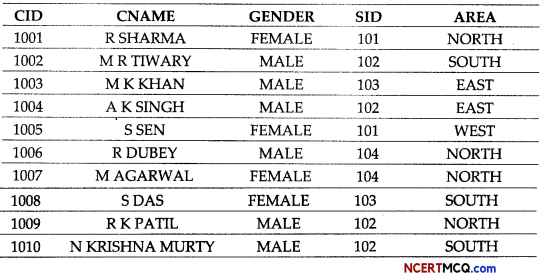(i) SELECT COUNT(*). GENDER FROM CUSTOMER GROUP BY GENDER: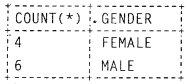(ii) SELECT CNAME FROM CUSTOMER WHERE CNAME LIKE ‘L%’;
No rows selected

(iii) SELECT DISTINCT AREA FROM CUSTOMER;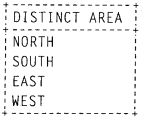(iv) SELECT CID, CNAME FROM CUSTOMER WHERE SID = 103;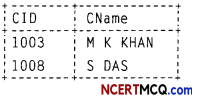Question 6.
(i) Write any two TCL commands.
COMMIT, ROLLBACK

(ii) Write the syntax to create a database in MySQL.
CREATE DATABASE <database_name>;Question 7.
Write the code using Python to create a table Product in database Inventory with following fields

 Fields Datatype PID varchar(5) PName char(30) Price float Rank varchar(2)

Or
Write the code to create the connection in which database’s name is Python, name of host, user and password can taken by user. Also, print that connection.

import mysql .connector
mycon = mysql.connector.connect (host = “localhost”, user = "system”, passwd = "hel1o”, database = “Inventory”)
cur = mycon.cursor( )
db = cur.execute(“CREATE TABLE Product(PID varchar(5) Primary key,
PName char (30),
Price float,
Rank varchar(2)))”
mycon.close( ) ,

Or

import mysql.connector
mycon = mysql.connector.connect(
host = “local host”,
user = "test”,
passwd = “testData",
database = “Python”)
print(mycon)

Section – B
(Each question carries 3 Marks)

Question 8.
Write the rules that are used while converting an infix expression to postfix using stack.
Or
Write the algorithm for push in a static stack. Also, write a function popdata() with a list of student names as parameter and display only those names that are of length more than 10.
Read all the symbols one by one from left to right in the given Infix Expression.

• If the reading symbol is operand, then directly print it to the result (Output).
• If the reading symbol is left parenthesis then Push it onto the Stack.
• If the reading symbol is right parenthesis’)’, then Pop all the contents of stack until respective left parenthesis is popped and print each popped symbol to the result.
• If the reading symbol is operator (+,-,*,/ etc.), then Push it on to the Stack. However, first pop the operators which are already on the stack that have higher or equal precedence than current operator and print them to the result.

Or

Algorithm

If top= MAX-1
Display “Stack Overflow”
Exit
Else
Top=top+l
Stack[Top]=data
Stop

Function

def popdata(Studnames):
size=len(Studnames)-1
while size>=0:
if len(Studnames[size]>10):
print(Studnames[size])
size- =1Question 9.
(i) Name any two operators used in queries.
(a) Arithmetic operators
(b) Comparison operators

(ii) What are the functions of ALTER TABLE command?
The main functions of ALTER TABLE command are
(b) Change the column definition of a column.
(d) Rename a column.

Question 10.
Consider the table MobileStock with following fields
M_Id, M_Name, M_Qty, M_Supplier
Write the Python code to fetch all records with fields M_Id, M_Name and M_Supplier from database Mobile.

import mysql.connector as mydb
mycon = mydb.connect (host = “localhost”, user = “root”, passwd = “system”, database = "Mobi1e”)
cursor = mycon.cursor()
sql = “SELECT M Id, M_Name,
M_Supplier FROM MobileStock”
try:
cursor.execute(sql)
display = cursor.fetchal1()
for i in display:
print(i)
except:
mycon.rollback()
mycon.close()

Section – C
(Each question carries A Marks)

Question 11.
Consider the following tables. Write SQL commands for the statements (i) to (iv).
Table : SENDER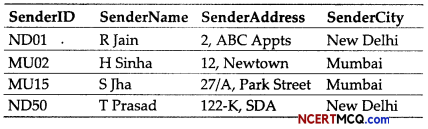Table : RECIPIENT(i) To display the names of all SENDERS from Mumbai.
SELECT SenderName FROM SENDER
WHERE SenderCity = ‘Mumbai’;

(ii) To display the RecID, SenderName, Sender Address, RecName, Rec Address for 5 every RECIPIENT.
SELECT RecID, SenderName,
FROM RECIPIENT, SENDER WHERE
RECIPIENT.SenderlD =
SENDER.SenderlD;

(iii) To display RECIPIENT details in ascending order of RecName.
SELECT * FROM RECIPIENT ORDER BY RecName;

(iv) To display number of RECIPIENTS from each City. ;
SELECT COUNT(*) AS “No. of Recipients”, RecCity FROM RECIPIENT
GROUP BY RecCity;Question 12.
(i) Explain two problems that can occur during transmission of data.
Or
Write down any two points of differences among LAN, MAN and WAN.
Two problems that can occur during transmission of data are as follows
(a) Crosstalk Disturbance caused by the electric or magnetic fields of one signal in an adjacent signal.
(b) Attenuation During transmission, the signal strength is reduced, this phenomenon is called attenuation.

Or

Two major points of differences among LAN, MAN and WAN are as follows:(ii) Define tree topology. Also, write down its two limitations.
A tree topology is an extension and variation of bus topology. Its basic structure is like an inverted tree, where the root acts as a server.

Limitations:
(a) Long cables are required for this kind of topologies.
(b) There is the dependence on the root node.

Question 13.
Quick Learn University is setting up its academic blocks at Prayag Nagar and planning to set up a network. The university has three academic blocks and one human resource centre as shown in the diagram below: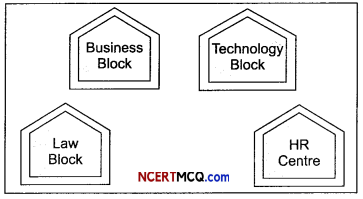Centre to centre distance between various blocks/centre is as follows:

 Law Block to Business Block 40 m Law Block to Technology Block 80 m Law Block to HR Centre 105 m Business Block to Technology Block 30 m Business Block to HR Centre 35 m Technology Block to HR Centre 15 m

Number of computers in each of the blocks/centre are as follows:

 Law Block 15 Technology Block 40 HR Centre 115 Business Block 25

(i) Suggest the most suitable place (i.e. block/centre) to install the server of this university with a suitable reason.